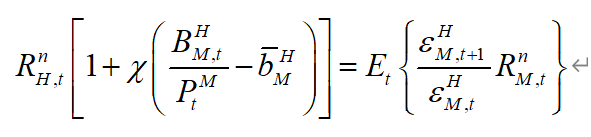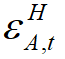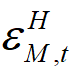# A question of three-country model

Hi guys, I have a question about the relationship of the three-country model. The question may be a little complicated but I really really need help, so thank you for your patience. Here it is.
There are three countries: H M and A.
For H, it has two UIPCs, which are(1) and(2)
H uses PEG poicy to A, wihch is=0.
(Rn_H, Rn_M and Rn_A are the nominal interest rate of H, M and A. ε_HM is the nominal exchage rate of H and A, which is the price of currency M measured by currency H. ε_HA is similar.is portfolio adjustment costs and B_HM is the bond of M bought by H, B_HA is the bond of A bought by H.)
For M, it also has two UIPCs, which are

andM uses Talor rules policy, which is
(note that:is the capital outflow shock, as said by Gentler 2007: External Constraints on Monetary Policy and the Financial Accelerator, I want to see the effects of capital ouflow to H sand M.)
So, here is my question. If there is a positive capital outflow shock, when meansincreases, then M will suffer a currency devaluation (that is,will increase andwill decrease), so from (1) we can see that Rn_H and B_HM will increase, and from (2) we can see that B_HA will decrease. These are the results of my IRFs. But, It is right?
For me, It looks like that, as the currency of M become cheaper, the capital of H still flows into M more than before. Does this match reality? (Besides, I found the M buys more bonds of A, while A buys more bonds of H, and at last, H buys more bonds of M, they have formed a circulation!)